Offset, Scaled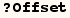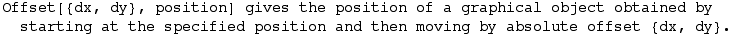In the next cell pnt1 is a red point at {1,0.025}.  The graphic made also shows pnt2, a blue point 20 printer points to the right and 10 printer points above {1,0.025}.  Notice distances in printer points aren't related to the scale on the axes.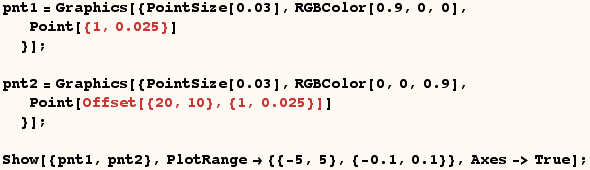The remaining examples used Offset and Scaled.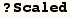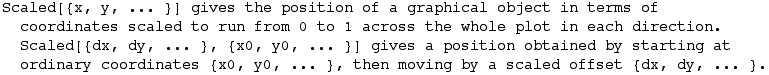In the next cell pnt1 is a red point positioned such that the distance from the left edge of the graphic is 10% of the graphic width, and and the distance from the bottom of the graphic is 5% of the graphic height. Also shown is pnt2, a blue point 20 printer points to the right and 10 printer points above pnt1.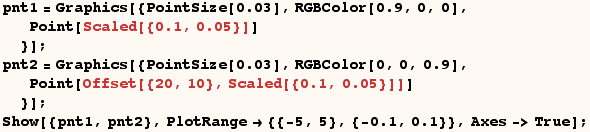In the next cell pnt1 is a red point at {1,0.025}.  Also shown is pnt2, a blue point at the position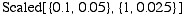which is to the right of pnt1 by 10% of the graphic width, and above pnt1 by 5% of the graphic height.  In addition pnt3 is shown which is a green point 10 printer points to the right of pnt2, and 20 printer points higher than pnt2.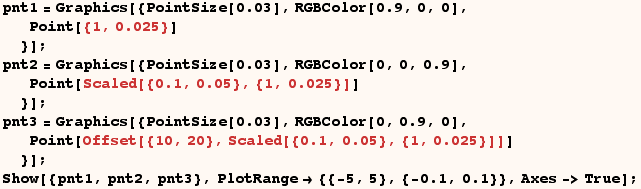A practical use of Offset is given in the next cell where it's used to specify the position of some text.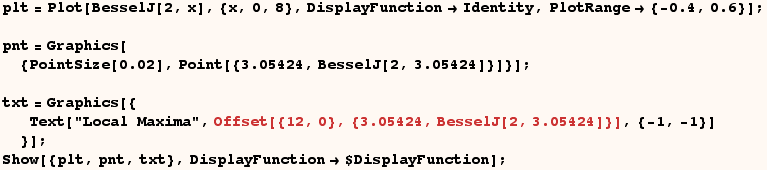Created by Mathematica  (May 16, 2004)

Back to Ted’s Tricks index page